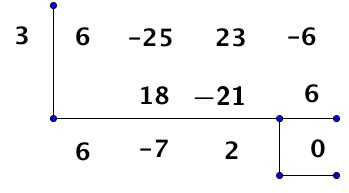# POLQ 3 | Lesson 2 | Practice (Rational Roots) Solutions

1. Factors of 6 are (leading coefficient and constant) : $$\pm {1}, \pm {2}, \pm {3}, \pm {6}$$
2. So all the possible roots $$\pm {1}, \pm{\dfrac{1}{2}}, \pm{\dfrac{1}{3}}, \pm{\dfrac{1}{6}}, \pm{\dfrac{2}{3}}, \pm {3}, \pm{6}$$

1. Factors of 9 are:  $$\pm {1}, \pm{3}, \pm{9}$$
2. Factors of 4 are: $$\pm { 1}, \pm {2}, \pm {4}$$

So the possible roots are:  $$\pm{1}, \pm{\dfrac{1}{2}}, \pm{\dfrac{1}{4}}, \pm{\dfrac{3}{2}}, \pm{\dfrac{3}{4}},\pm{9}\,\pm{\dfrac{9}{2}}, \pm{\dfrac{9}{4}}$$

1. Factors of 4 are: $$\pm { 1}, \pm {2}, \pm {4}$$
2. Factors of 6 are:  $$\pm {1}, \pm {2}, \pm {3}, \pm {6}$$

So the possible roots are $$\pm { 1}, \pm {2}, \pm {4}, \pm{\dfrac{1}{2}}, \pm{\dfrac{1}{3}}, \pm{\dfrac{1}{6}},\pm{\dfrac{2}{3}},\pm{\dfrac{4}{3}}$$

1. Find the roots for problem 1
2. Try 3 as a root and it works as shown below because it has a remainder of 0.Factoring the quadratic:  $$6x^2-7x+2 = (2x-1)(3x-2)$$

Therefore the roots of the equation are:  $$3$$,$$\dfrac{1}{2}$$ and $$\dfrac{2}{3}$$